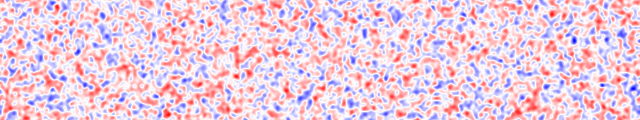## Stochastic Processes and Complex Systems

### Faculty involved

• Polynomial Chaos expansions
• Uncertainty Quantification
• Design and Optimization under Uncertainty
• Data Assimilation
• Stochastic modeling
• Mathematical Finance

With applications to:

• Climate science
• Biomedical Applications
• Material sciences
• Inverse problems
• Fluid dynamics
• Electromagnetic and optic problems
• Optimal design of structures
• Neuroscience
• Physiology

Other groups within the Mathematics department that do stochastic calculations are the Probability and Statistics group and the Mathematical Biology groupFlow past a circular cylinder with a uniform inflow (left) and a non-uniform Gaussian random inflow (right). (from “Modeling uncertainty in flow simulations via generalized polynomial chaos”, D. Xiu, G. E. Karniadiakis).Although the field above is random, it does have certain recognizable properties such as amplitude and size of fluctuations. Such random fields can be used to model fluctuations in e.g. material or physical properties (one example is small fluctuations of the speed of waves in the earth due to small inhomogeneities, such as pebbles).

Webmaster: Fernando Guevara Vasquez. (Created by Yekaterina Epshteyn and FGV)